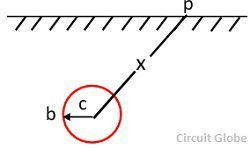The potential gradient in a power system is defined as the rate of change of electric potential with respect to the distance from the base of the electrical structure. The resistance of the earth electrode is not concentrated at one point, but it is distributed over the soil around the electrode. When a fault current flows to ground, it results in a potential gradient around the electrode. This may be explained analytically as below

Consider that the base of the structure through which fault current is flowing to ground is a hemisphere of radius b as shown in fig below,ex – electrical field strength at a distance x in V/m
ρ – resistivity in  ohm-meters
If – fault current in amperes
x – distance from the surface of the hemisphere in metersIf a curve between the fall of potential with distance from the base of the structure is plotted, it is observed that the potential difference is quite definite near the electrode and the fault. The magnitude of potential gradient depends on the resistivity and fault current. If the magnitude of the potential gradient is high then it may affect the person by step and touch potential.

For safety purpose, the earthing system should be provided such that the potential difference due to the fault may not prove to be dangerous to the person approaching that electrode or while touching that structure.